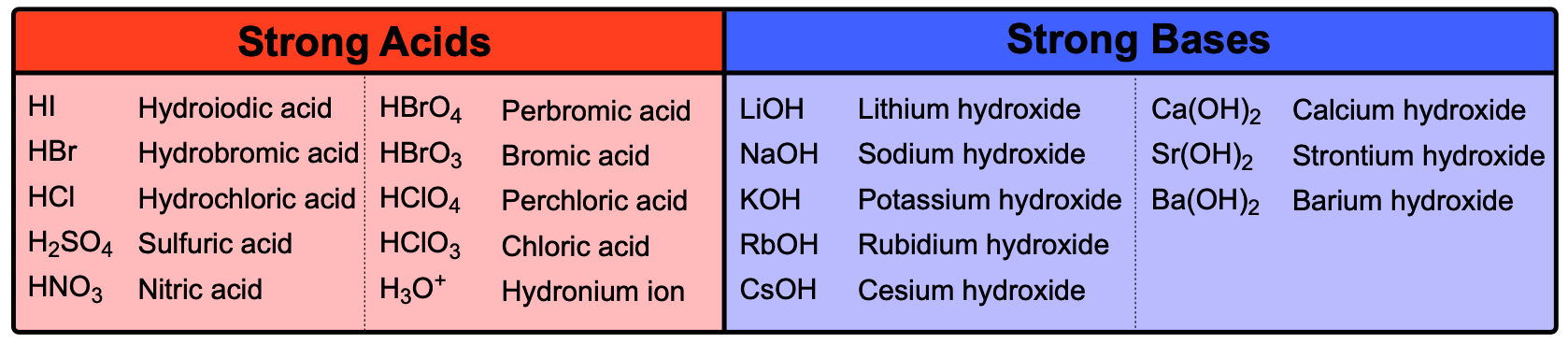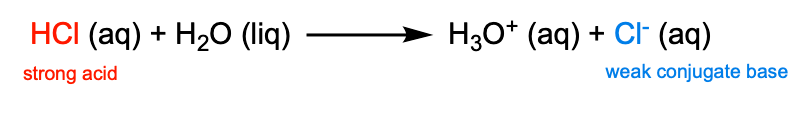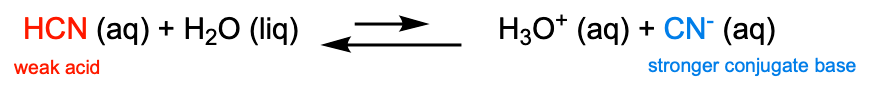Clutch Prep is now a part of Pearson
Ch.10 Acids and BasesWorksheetSee all chapters

# Acid and Base Strength

See all sections
Sections
Acid-Base Introduction
Arrhenius Acid and Base
Bronsted Lowry Acid and Base
Acid and Base Strength
Ka and Kb
The pH Scale
Auto-Ionization
pH of Strong Acids & Bases
Acid-Base Equivalents
Acid-Base Reactions
Gas Evolution Equations (Simplified)
Ionic Salts (Simplified)
Buffers
Henderson-Hasselbalch Equation
Strong Acid Strong Base Titrations (Simplified)

Strong Acids and Strong Bases are classified as Strong Electrolytes. While Weak Acids and Bases are Weak Electrolytes.

###### Understanding Acid and Base Strength

Concept #1: Strong vs Weak Acids

Concept #2: Strong vs Weak BasesExample #1: The following represent aqueous acid solutions. Identify the strong acid, weak acid and weakest acid.

Concept #3: Strength of Conjugate Bases

Stronger the acid, weaker the conjugate base.Concept #4: Strength of Conjugate Acids

Weaker the acid, stronger the conjugate base.Example #2: Which of the following acids have relatively strong conjugate bases?

Practice: Which of the following is the strongest base?

Practice: Which of the following bases will have the weakest conjugate acid?

Practice: Which of the following aqueous species will contain mostly reactants?

Practice: Determine [OH] in each base solution. If the base is weak, indicate the value that [OH] is less than.

Practice: Predict the direction of the following reaction:

HC2H3O2 (aq)  +  H2O (liq)  ______________   H3O+ (aq)  +  C2H3O2 (aq)